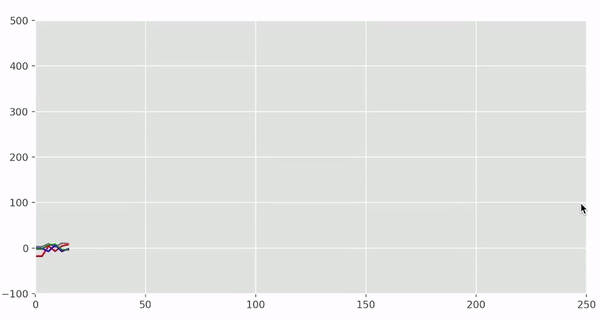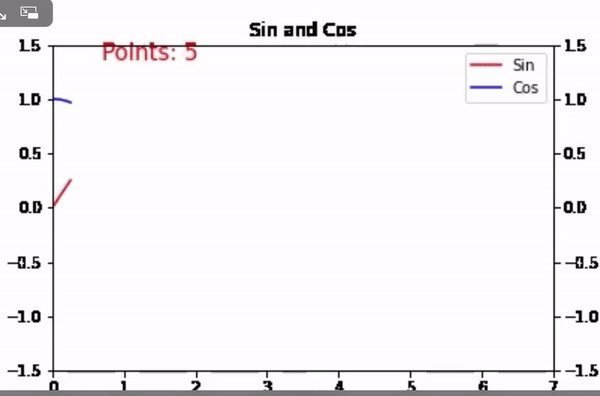Related Articles
Matplotlib – Animate Multiple Lines
• Difficulty Level : Medium
• Last Updated : 30 Apr, 2021

In this article, we are going to learn about how to make an animated chart of multiple lines using matplotlib. Animating the charts can make things more attractive and also help others to visualize the data more appropriately rather than static charts. Animations make even more sense when we are working with projects(stock markets, ECG Anomaly Detection, Internet Traffic Forecasting) that depicts the time series data.

The matplotlib.animation.FuncAnimation class is used to make animation calls recursively. You must store the created Animation in a variable that lives as long as the animation should run. Otherwise, the Animation object will be garbage-collected and the animation stops.

Syntax: class matplotlib.animation.FuncAnimation(fig, func, frames=None, init_func=None, fargs=None, save_count=None, *, cache_frame_data=True, **kwargs)

Example 1:

For matplotlib there are two important modules we need primarily: pyplot and animation(Funcanimation). Below is a step-wise approach on how to animate lines in matplotlib. We are going to make our first example with 4 manually built plots using the random numbers in a certain range.

• Import all necessary libraries for creating charts and animation.

## Python3

 `# importing all necesaary libraries``import` `random``import` `matplotlib``from` `matplotlib ``import` `animation``import` `matplotlib.pyplot as plt``from` `matplotlib.animation ``import` `FuncAnimation``%``matplotlib qt`
• Now make 4 different sets of y i.e y1,y2,y3,y4  which are going to share with the same x-axis values. While taking the random values we are going to divide each random value because these will help us to get different exponential lines.

## Python3

 `# add random points for each line``l1 ``=` `[random.randint(``-``20``, ``4``)``+``(points``*``*``1.88``)``/``(random.randint(``13``, ``14``))``      ``for` `points ``in` `range``(``0``, ``160``, ``2``)]``l2 ``=` `[random.randint(``0``, ``9``)``+``(points``*``*``1.9``)``/``(random.randint(``9``, ``11``))``      ``for` `points ``in` `range``(``0``, ``160``, ``2``)]``l3 ``=` `[random.randint(``-``10``, ``10``)``-``(points``*``*``1.4``)``/``(random.randint(``9``, ``12``))``      ``for` `points ``in` `range``(``0``, ``160``, ``2``)]``l4 ``=` `[random.randint(``-``5``, ``10``)``-``(points``*``*``1.1``)``/``(random.randint(``7``, ``12``))``      ``for` `points ``in` `range``(``0``, ``160``, ``2``)]`
• Now use itertools to iterate. This module works fast, it is a memory-efficient tool that is used either by itself or in combination to form iterator algebra. You can also use for loop to iterate just create one list and start storing the variables of y w.r.t x.

## Python3

 `from` `itertools ``import` `count``myvar ``=` `count(``0``, ``3``)`
• Create 3 extra empty lists for new lines (y2 ,y3, and y4) total of 5 empty lists when we include x1 and y1. Inside the animation function, we will fill those containers at each iteration step. In each iteration single frame created animation. Also, add colors for 4 different lines.

## Python3

 `# subplots() function you can draw ``# multiple plots in one figure``fig, axes ``=` `plt.subplots(nrows``=``1``, ncols``=``1``, figsize``=``(``10``, ``5``))`` ` `# set limit for x and y axis``axes.set_ylim(``-``100``, ``500``)``axes.set_xlim(``0``, ``250``)`` ` `# style for plotting line``plt.style.use(``"ggplot"``)`` ` `# create 5 list to get store element``# after every iteration``x1, y1, y2, y3, y4 ``=` `[], [], [], [], []``myvar ``=` `count(``0``, ``3``)`` ` `def` `animate(i):``    ``x1.append(``next``(myvar))``    ``y1.append((l1[i]))``    ``y2.append((l2[i]))``    ``y3.append((l3[i]))``    ``y4.append((l4[i]))`` ` `    ``axes.plot(x1, y1, color``=``"red"``)``    ``axes.plot(x1, y2, color``=``"gray"``)``    ``axes.plot(x1, y3, color``=``"blue"``)``    ``axes.plot(x1, y4, color``=``"green"``)`` ` ` ` `# set ani variable to call the``# function recursively``anim ``=` `FuncAnimation(fig, animate, interval``=``30``)`

Below is the complete program:

## Python3

 `# importing all necesaary libraries``from` `itertools ``import` `count``import` `random``import` `matplotlib``from` `matplotlib ``import` `animation``import` `matplotlib.pyplot as plt``from` `matplotlib.animation ``import` `FuncAnimation``%``matplotlib qt`` ` `# add random points for each line``l1 ``=` `[random.randint(``-``20``, ``4``)``+``(points``*``*``1.88``)``/``(random.randint(``13``, ``14``))``      ``for` `points ``in` `range``(``0``, ``160``, ``2``)]``l2 ``=` `[random.randint(``0``, ``9``)``+``(points``*``*``1.9``)``/``(random.randint(``9``, ``11``))``      ``for` `points ``in` `range``(``0``, ``160``, ``2``)]``l3 ``=` `[random.randint(``-``10``, ``10``)``-``(points``*``*``1.4``)``/``(random.randint(``9``, ``12``))``      ``for` `points ``in` `range``(``0``, ``160``, ``2``)]``l4 ``=` `[random.randint(``-``5``, ``10``)``-``(points``*``*``1.1``)``/``(random.randint(``7``, ``12``))``      ``for` `points ``in` `range``(``0``, ``160``, ``2``)]`` ` `myvar ``=` `count(``0``, ``3``)`` ` `# subplots() function you can draw ``# multiple plots in one figure``fig, axes ``=` `plt.subplots(nrows``=``1``, ncols``=``1``, figsize``=``(``10``, ``5``))`` ` `# set limit for x and y axis``axes.set_ylim(``-``100``, ``500``)``axes.set_xlim(``0``, ``250``)`` ` `# style for plotting line``plt.style.use(``"ggplot"``)`` ` `# create 5 list to get store element ``# after every iteration``x1, y1, y2, y3, y4 ``=` `[], [], [], [], []``myvar ``=` `count(``0``, ``3``)`` ` `def` `animate(i):``    ``x1.append(``next``(myvar))``    ``y1.append((l1[i]))``    ``y2.append((l2[i]))``    ``y3.append((l3[i]))``    ``y4.append((l4[i]))`` ` `    ``axes.plot(x1, y1, color``=``"red"``)``    ``axes.plot(x1, y2, color``=``"gray"``)``    ``axes.plot(x1, y3, color``=``"blue"``)``    ``axes.plot(x1, y4, color``=``"green"``)`` ` ` ` `# set ani variable to call the ``# function recursively``anim ``=` `FuncAnimation(fig, animate, interval``=``30``)`

Output :Example 2:

Here is another example to animate multiple lines in matplotlib.

• Import all necessary Libraries.

## Python3

 `# import modules``import` `numpy as np``import` `matplotlib``import` `matplotlib.pyplot as plt``import` `matplotlib.animation as animation`
• Create a function update line to get a new value for each iteration.

## Python3

 `def` `updateline(num, data, line1, data2, line2):``    ``line1.set_data(data[..., :num])``    ``line2.set_data(data2[..., :num])``     ` `    ``time_text.set_text(``"Points: %.0f"` `%` `int``(num))``     ` `    ``return` `line1, line2`` ` ` ` `# generating data of 100 elements``# each for line 1``x ``=` `np.linspace(``0``, ``2``*``np.pi, ``100``)``y ``=` `np.sin(x)``data ``=` `np.array([x, y])`` ` `# generating data of 100 elements ``# each for line 2``x2 ``=` `np.linspace(``0``, ``2``*``np.pi, ``100``)``y2 ``=` `np.cos(x2)``data2 ``=` `np.array([x2, y2])`` ` `# setup the formating for moving files``Writer ``=` `animation.writers[``'ffmpeg'``]``Writer ``=` `Writer(fps``=``10``, metadata``=``dict``(artist``=``"Me"``), bitrate``=``-``1``)`` ` ` ` `fig ``=` `plt.figure()``ax ``=` `fig.add_subplot(``111``)``l, ``=` `ax.plot([], [], ``'r-'``, label``=``"Sin"``)``ax2 ``=` `ax.twinx()``k ``=` `ax2.plot([], [], ``'b-'``, label``=``"Cos"``)[``0``]`` ` `ax.legend([l, k], [l.get_label(), k.get_label()], loc``=``0``)`` ` `ax.set_xlabel(``"X"``)`` ` `# axis 1``ax.set_ylim(``-``1.5``, ``1.5``)``ax.set_xlim(``0``, ``7``)`` ` `# axis 2``ax2.set_ylim(``-``1.5``, ``1.5``)``ax2.set_xlim(``0``, ``7``)`` ` `plt.title(``'Sin and Cos'``)``time_text ``=` `ax.text(``0.1``, ``0.95``, "", transform``=``ax.transAxes,``                    ``fontsize``=``15``, color``=``'red'``)`
• Save mp4 file [By default files saved in your present directory].

## Python3

 `# set line_animation variable to call``# the function recursively``line_animation ``=` `animation.FuncAnimation(``    ``fig, updateline, frames``=``100``, fargs``=``(data, l, data2, k))`` ` `line_animation.save(``"lines.mp4"``, writer``=``Writer)`

Below is the complete program:

## Python3

 `# import required modules``import` `numpy as np``import` `matplotlib``import` `matplotlib.pyplot as plt``import` `matplotlib.animation as animation`` ` ` ` `def` `updateline(num, data, line1, data2, line2):``    ``line1.set_data(data[..., :num])``    ``line2.set_data(data2[..., :num])``     ` `    ``time_text.set_text(``"Points: %.0f"` `%` `int``(num))``     ` `    ``return` `line1, line2`` ` ` ` `# generating data of 100 elements``# each for line 1``x ``=` `np.linspace(``0``, ``2``*``np.pi, ``100``)``y ``=` `np.sin(x)``data ``=` `np.array([x, y])`` ` `# generating data of 100 elements``# each for line 2``x2 ``=` `np.linspace(``0``, ``2``*``np.pi, ``100``)``y2 ``=` `np.cos(x2)``data2 ``=` `np.array([x2, y2])`` ` `# setup the formating for moving files``Writer ``=` `animation.writers[``'ffmpeg'``]``Writer ``=` `Writer(fps``=``10``, metadata``=``dict``(artist``=``"Me"``), bitrate``=``-``1``)`` ` ` ` `fig ``=` `plt.figure()``ax ``=` `fig.add_subplot(``111``)``l, ``=` `ax.plot([], [], ``'r-'``, label``=``"Sin"``)``ax2 ``=` `ax.twinx()``k ``=` `ax2.plot([], [], ``'b-'``, label``=``"Cos"``)[``0``]`` ` `ax.legend([l, k], [l.get_label(), k.get_label()], loc``=``0``)`` ` `ax.set_xlabel(``"X"``)`` ` `# axis 1``ax.set_ylim(``-``1.5``, ``1.5``)``ax.set_xlim(``0``, ``7``)`` ` `# axis 2``ax2.set_ylim(``-``1.5``, ``1.5``)``ax2.set_xlim(``0``, ``7``)`` ` `plt.title(``'Sin and Cos'``)``time_text ``=` `ax.text(``0.1``, ``0.95``, "", transform``=``ax.transAxes,``                    ``fontsize``=``15``, color``=``'red'``)`` ` `# set line_animation variable to call ``# the function recursively``line_animation ``=` `animation.FuncAnimation(``    ``fig, updateline, frames``=``100``, fargs``=``(data, l, data2, k))``line_animation.save(``"lines.mp4"``, writer``=``Writer)`

Output :Attention geek! Strengthen your foundations with the Python Programming Foundation Course and learn the basics.

To begin with, your interview preparations Enhance your Data Structures concepts with the Python DS Course. And to begin with your Machine Learning Journey, join the Machine Learning – Basic Level Course

My Personal Notes arrow_drop_up